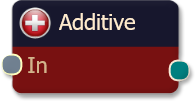#### Risk Disclosure

Futures, foreign currency and options trading contains substantial risk and is not for every investor. An investor could potentially lose all or more than the initial investment. Risk capital is money that can be lost without jeopardizing ones financial security or lifestyle. Only risk capital should be used for trading and only those with sufficient risk capital should consider trading. Past performance is not necessarily indicative of future results. View full risk disclosure.The additive logic node is a simple node that simply adds the input values together to produce the output. This node type, while not necessarily a fuzzy logic function, can be used to emulate trading systems that run on a point scoring basis. For instance suppose you have designed a trading system that had 4 separate criteria. Your system resembles the following:

1. If criteria 1 is true add 1 point
2. If criteria 2 is true add 2 points
3. If criteria 3 is true add 2 points
4. If criteria 4 is true add 3 points

After examining all the criteria, if you have accumulated 5 or more points, take the trade.

The additive logic node is designed to emulate systems like this.

## Example

To implement the example, you would start by adding all 4 solvers, one for each criteria. Remember since BloodHound works on a scale from 0 to 1, we have to normalize the point system to work on this scale. The easiest way to think of this is in percentages of your total goal. If your goal is to accumulate 5 points, we will now express the points for each criteria as some percentage of 5, like the following:

1. If criteria 1 is true add 0.20 points (1/5)
2. If criteria 2 is true add 0.40 points (2/5)
3. If criteria 3 is true add 0.40 points (2/5)
4. If criteria 4 is true add 0.60 points (3/5)

Now, if you examine the criteria and your total is 1.0 or more you would take the trade. You will notice that this is the equivalent system to the example defined above, just that the points have been normalized for a range of 1.0 (instead of 5).

Now add all the solver nodes to the logic template along with on Additive logic node. Connect all the solvers nodes output to the inputs on the Additive logic node. Finally we have one more important step, and that is to contain the output ranges of the solvers. Click the Additive logic node to select it. You will now notice that all the solvers added to this node have weight sliders on the right hand pane.

Manipulate the weight slider for criteria 1 so that the range goes from 0 to 0.20 (instead of 1.0). Likewise, change the weight slider for criteria 2 to range 0 to 0.40. Once you have completed the last two criteria in a similar fashion you are done.